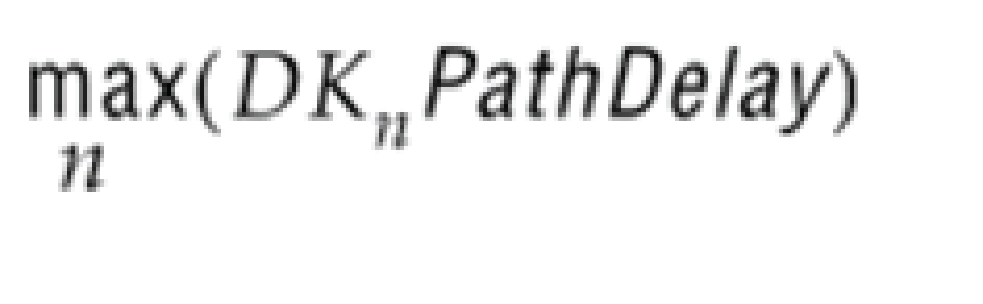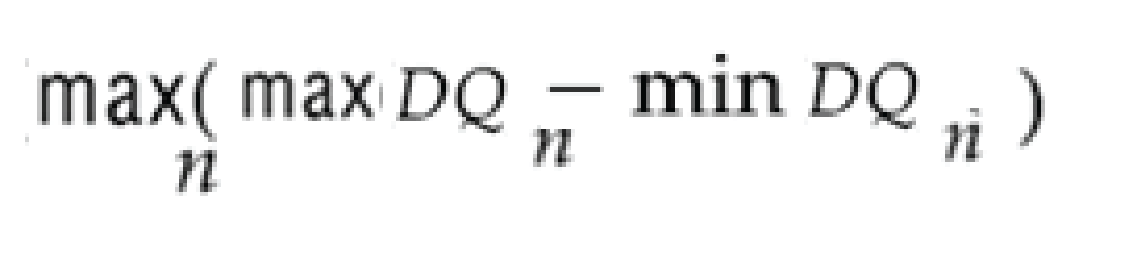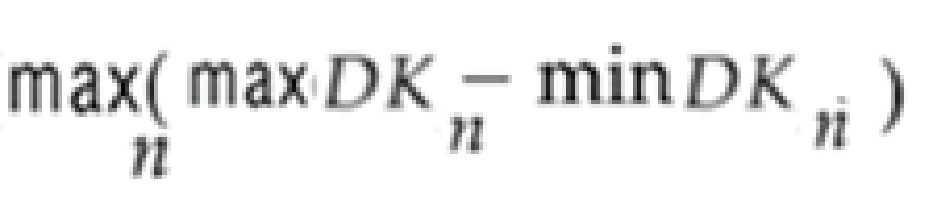ID 683385
Date 5/08/2017
Public

## 7.4.9.4. Equations for RLDRAM 3 Board Skew Parameters

Table 290.  Parameter Equations
Parameter Description/Equation
Maximum CK delay to device The delay of the longest CK trace from the FPGA to any device.where n is the number of memory clocks. For example, the maximum CK delay for two pairs of memory clocks is expressed by the following equation:Maximum DK delay to device The delay of the longest DK trace from the FPGA to any device.where n is the number of DK. For example, the maximum DK delay for two DK is expressed by the following equation:Average delay difference between DK and CK

The average delay difference between the DK signals and the CK signal, calculated by averaging the longest and smallest DK delay minus the CK delay. Positive values represent DK signals that are longer than CK signals and negative values represent DK signals that are shorter than CK signals. The Quartus Prime software uses this skew to optimize the delay of the DK signals to have appropriate setup and hold margins.where n is the number of memory clocks and m is the number of DK.
Maximum system skew within address/command busThe largest skew between the address and command signals. Enter combined board and package skew.
Average delay difference between address/command and CK The average delay difference between the address and command signals and the CK signal, calculated by averaging the longest and smallest Address/Command signal delay minus the CK delay. Positive values represent address and command signals that are longer than CK signals and negative values represent address and command signals that are shorter than CK signals. The Quartus Prime software uses this skew to optimize the delay of the address and command signals to have appropriate setup and hold margins.Maximum board skew within QK group

The largest skew between all DQ and DM pins in a QK group. Enter your board skew only. Package skew will be calculated automatically, based on the memory interface configuration, and added to this value. This value affects the read capture and write margins.where n is the number of DQ.
Maximum skew between DK groups The largest skew between DK signals in different DK groups.where n is the number of DQ.